Courses

# Synchronous Machine Armature Windings - 2 Notes | EduRev

## Electrical Engineering (EE) : Synchronous Machine Armature Windings - 2 Notes | EduRev

The document Synchronous Machine Armature Windings - 2 Notes | EduRev is a part of the Electrical Engineering (EE) Course Electrical Machines for Electrical Engg..
All you need of Electrical Engineering (EE) at this link: Electrical Engineering (EE)

Frequency of an A.C. Synchronous Generator
Commercial ac synchronous generators have many poles and may rotate at various speeds, either as alternators or as synchronous or induction motors.Eqn. 13 was derived for a two-pole device in which the generated EMF in the stationary armature winding changes direction every half-revolution of the two-pole rotor. One complete revolution will produce one complete positive and negative pulse each cycle. The frequency in cycles per second (Hz) will, as stated previously, depend directly on the speed or number of revolutions per second (rpm/60) of the rotating ﬁeld.

If the ac synchronous generator has multiple poles (having, say, two, four, six, or eight poles...), then for a speed of one revolution per second (1 rpm/60), the frequency per revolution will be one, two, three, or four ..., cycles per revolution, respectively. The frequency per revolution, is therefore, equal to the number of pairs of poles. Since the frequency depends directly on the speed (rpm/60) and also on the number of pairs of poles (P/2), we may combine these into a single equation in which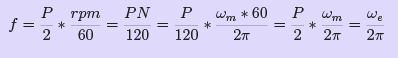(18)
where
P is the number of poles
N is the speed in rpm (rev/min)
f is. the frequency in hertz
ωe is the speed electrical radians per second.

Constructional Details of Rotor

As stated earlier the ﬁeld windings are provided in the rotor or the rotating member of the synchronous machine. Basically there are two general classiﬁcations for large 3 phase synchronous generators ——cylindrical rotor and salient-pole rotor - .

The cylindrical-rotor construction is peculiar to synchronous generators driven by steam turbines and which are also known as turbo alternators or turbine generators. Steam turbines operate at relatively high speeds, 1500 and 3000 rpm being common for 50 Hz, accounting for the cylindrical-rotor construction, which because of its compactness readily withstands the centrifugal forces developed in the large sizes at those speeds. In addition the smoothness of the rotor contour makes for reduced windage losses and for quiet operation.

Salient-pole rotors are used in low-speed synchronous generators such as those driven by water wheels. They are also used in synchronous motors. Because of their low speeds salient-pole generators require a large number of poles as, for example, 60 poles for a 100-rpm 50 Hz generator.

Fig. 14 illustrates two and four pole cylindrical rotors along with a developed view of the ﬁeld winding for one pair of poles. One pole and its associated ﬁeld coil of a salient-pole rotor is shown in ﬁg. 14.The stator slots in which the armature winding is embedded are not shown for reasons of simplicity. The approximate path taken by the ﬁeld ﬂux, not including leakage ﬂux, is indicated by the dashed lines in Fig. 14. The ﬁeld coils in Fig. 14 are represented by ﬁlaments but actually (except for the insulation between turns and between the coil sides and the slot) practically ﬁll the slot more nearly in keeping with ﬁg. 15.

The stepped curve in ﬁg. 15. represents the waveform of the mmf produced by the distributed ﬁeld winding if the slots are assumed to be completely ﬁlled by the copper in the coil sides instead of containing current ﬁlaments. The sinusoid indicated by the dashed line in ﬁg. 15 represents approximately the fundamental component of the mmf wave.

The air gap in cylindrical-rotor machines is practically of uniform length except for the slots in the rotor and in the stator, and when the eﬀect of the slots and the tangential component of H, which is quite small for the low ratio of air-gap length to the arc subtended by one pole in conventional machines, are neglected, the stepped mmf wave in ﬁg. 15 produces a ﬂux-density space wave in which the corners of the steps are rounded due to fringing. The ﬂux density wave form is therefore more nearly sinusoidal than the mmf waveform when the eﬀect of the slots is neglected. However, saturation of the iron in the region of maximum mmf tends to ﬂatten the top of the ﬂux-density wave.

Excitation Systems for Synchronous Machines

A number of arrangements for supplying direct current to the ﬁelds of synchronous machines have come into use. Adjustments in the ﬁeld current may be automatic or manual depending upon the complexity and the requirements of the power system to which the generator is connected.

Excitation systems are usually 125 V up to ratings of 50kW with higher voltages for the larger ratings. The usual source of power is a direct-connected exciter, motor- generator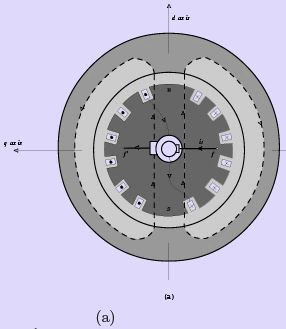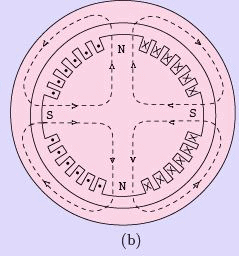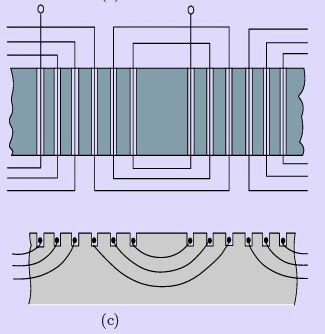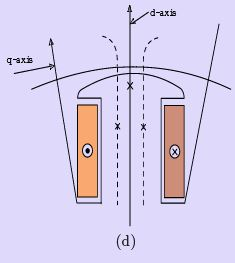Figure 14: Synchronous machines with stator slots and armature windings omitted (a)Twopole cylindrical rotor, (b) Four-pole cylindrical rotor, (c) Developed view of two pole cylindrical rotor ﬁeld structure, (d) Salient pole and ﬁeld coil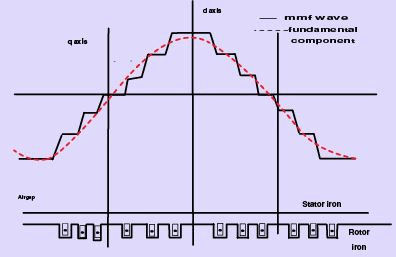Figure 15: Cylindrical rotor mmf wave and its fundamental of a synchronous machine

set, rectiﬁer, or battery. A common excitation system in which a conventional dc shunt generator mounted on the shaft of the synchronous machine furnishes the ﬁeld excitation is shown in Fig. 16. The output of the exciter (i.e., the ﬁeld current of the synchronous machine) is varied by adjusting the exciter ﬁeld rheostat. A somewhat more complex system that makes use of a pilot exciter—- a compound dc generator—- also mounted on the generator shaft, which in turn excites the ﬁeld of the main exciter, is shown in Fig. 16. This arrangement makes for greater rapidity of response, a feature that is important in the case of synchronous generators when there are disturbances on the system to which the generator is connected. In some installations a separate motor-driven exciter furnishes the excitation.
An induction motor is used instead of a synchronous motor because in a severe system disturbance a synchronous motor may pullout of synchronism with the system. In addition, a large ﬂywheel is used to carry the exciter through short periods of severely reduced system voltage.

Brushless Excitation System

The brushless excitation system eliminates the usual commutator, collector rings, and brushes. One arrangement in which a permanent magnet pilot exciter, an ac main exciter, and a rotating rectiﬁer are mounted on the same shaft as the ﬁeld of the ac turbogenerator is shown in Fig. 17. The permanent magnet pilot excitor has a stationary armature and a rotating permanent magnetic ﬁeld. It feeds 400 Hz, three-phase power to a regulator, which in turn supplies regulated dc power to the stationary ﬁeld of a rotating-armature ac exciter, The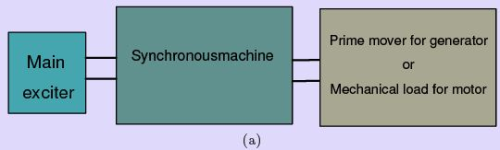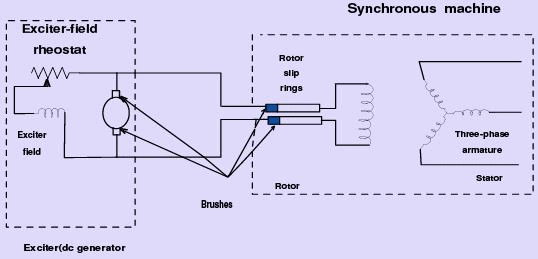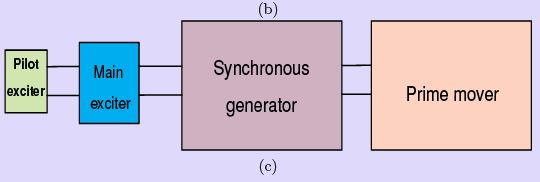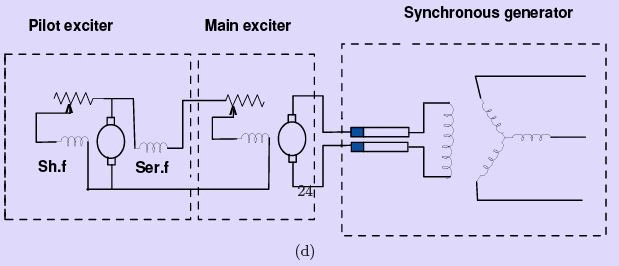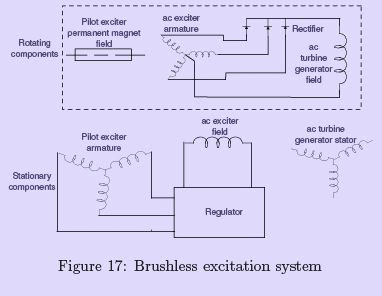Figure 17: Brushless excitation system

output of the ac exciter is rectiﬁed by diodes and delivered to the ﬁeld of the turbo generator.

Brush less excitation systems have been also used extensively in the much smaller generators employed in aircraft applications where reduced atmospheric pressure intensiﬁes problems of brush deterioration. Because of their mechanical simplicity, such systems lend themselves to military and other applications that involve moderate amounts of power.

The Action of the Synchronous Machine

Just like the DC generator, the behaviour of a Synchronous generator connected to an external load is not the same as at no-load. In order to understand the action of the Synchronous machine when it is loaded, let us take a look at the ﬂux distributions in the machine when the armature also carries a current. Unlike in the DC machine here the current peak and the emf peak will not occur in the same coil due to the eﬀect of the power factor (pf ) of the load. In other words the current and the induced emf will be at their peaks in the same coil only for upf loads. For zero power factor (zpf )(lagging) loads, the current reaches its peak in a coil which falls behind that coil wherein the induced emf is at its peak by nearly 90 electrical degrees or half a pole-pitch. Likewise for zero power factor (zpf )(leading) loads, the current reaches its peak in a coil which is ahead of that coil wherein the induced emf is at its peak by nearly 90 electrical degrees or half a pole-pitch. For simplicity, let us assume the resistance and leakage reactance of the stator windings to be negligible. Let us also assume the magnetic circuit to be linear i.e. the ﬂux in the magnetic circuit is deemed to be proportional to the resultant ampere-turns - in other words we assume that there is no saturation of the magnetic core. Thus the e.m.f. induced is the same as the terminal voltage, and the phase-angle between current and e.m.f. is determined only by the power factor (pf ) of the external load connected to the synchronous generator.

Armature Reaction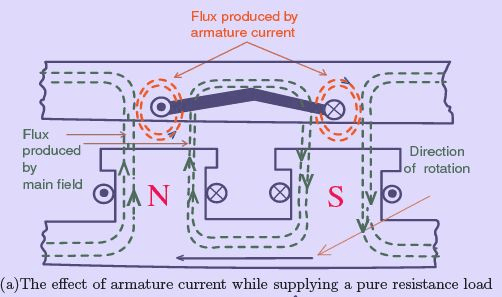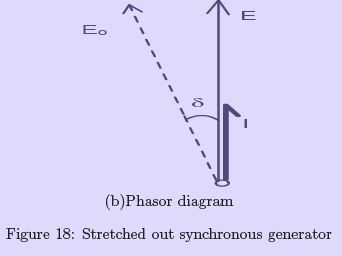In order to understand more clearly let us consider a sketch of a stretched-out synchronous machine shown in Fig. 18(a) which shows the development of a ﬁxed stator car-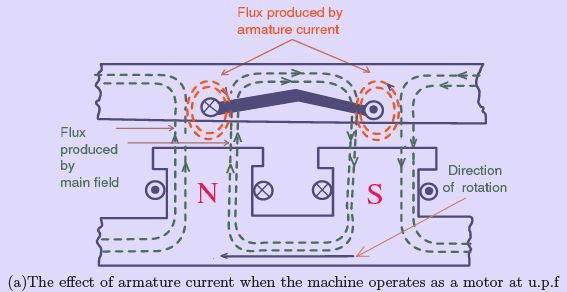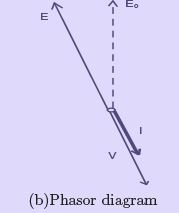Figure 19: Stretched out synchronous motor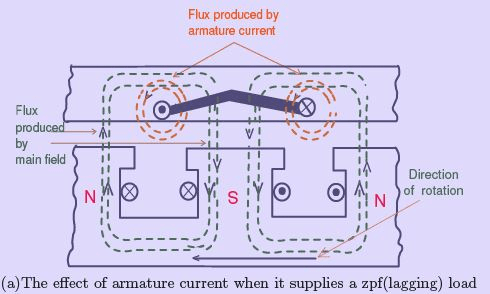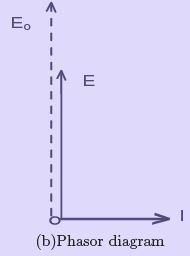Figure 20: Stretched out synchronous generator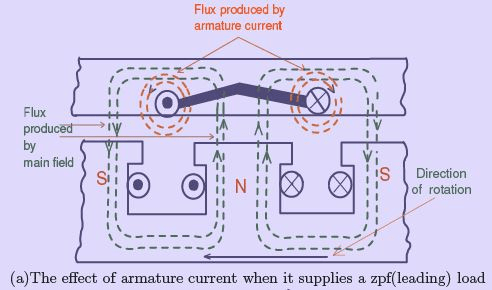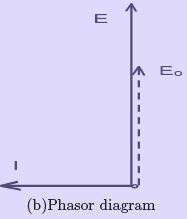Figure 21: Stretched out synchronous generator

rying armature windings, and a rotor carrying ﬁeld windings and capable of rotation within it. The directions of the currents and the ﬂux distribution are as shown in Fig. 18(a), when the emf induced in the stator coils is the maximum. The coil links no resultant ﬂux but is in the position of greatest rate of change of ﬂux. The coil position shown is also that for maximum current when the current is in phase with the voltage: i.e for a pure resistive load.
The current in the coil has no eﬀect on the total ﬂux per pole, but causes a strengthening on one side and a weakening on the other side of the pole shoes. Thus the armature conductors ﬁnd themselves in the circumstances illustrated in Fig. 19, and a torque is produced by the interaction of the main ﬂux φm with the current in the conductors. The torque thus produced is seen to be opposed to the direction of motion of the rotor - the force on the conductors is such as to push them to the left and by reaction to push the rotor to the right (as the armature coils are stationary). The rotor is rotated by a prime mover against this reaction, so that the electrical power, the product E I , is produced by virtue of the supply of a corresponding mechanical power. Thus it is evident from the distortion of the main ﬂux distribution that electrical energy is converted from mechanical energy and the machine operates as a generator. An unidirectional torque is maintained as the stator conductors cut N-Pole and S-Pole ﬂuxes alternately resulting in alternating emfs at a frequency equal to the number of pole-pairs passed per second and the currents also alternate with the emf.
The assumption that the conditions shown in Fig. 18(a) represent co-phasal emf and current is not quite true. The strengthening of the resultant ﬂux on the right of the poles and an equivalent amount of weakening on the left eﬀectively shift the main ﬁeld ﬂux axis against the direction of rotation, so that the actual e.m.f. E induced in the armature winding is an angle δ behind the position E0 that it would occupy if the ﬂux were undistorted as shown in the adjacent phasor diagram Fig. 18(b) pertaining to this condition of operation. Thus the eﬀect of a resistive (unit power factor (upf )) load connected to a synchronous generator is to shift the main ﬁeld ﬂux axis due to what is known as cross-magnetization.

The action of a synchronous machine operating as a motor at unit power factor (upf ) is shown in Fig. 19(a). Just like a DC motor, a synchronous motor also requires an externally-applied voltage V in order to circulate in it a current in opposition to the induced e.m.f. E. The coil is shown in the position of maximum induced emf and current, but the current is oppositely directed to that shown in Fig. 18(a). Again the m.m.f. of the coil does not aﬀect the total ﬂux in the common magnetic circuit, but distorts the distribution in such a way as to produce a torque in the same direction as the motion. The machine is a motor by virtue of the electrical input VI causing a torque in the direction of motion.
The ﬂux distortion causes a shift of the ﬂux axis across the poles, so that the actual e.m.f.
E is an angle δ ahead of the position E0 that it would occupy if the ﬂux were undistorted as shown in the adjacent phasor diagram Fig. 19(b), pertaining to this condition of operation.

Next let us consider this generator to be connected to a purely inductive load so that the current I in the coils lags behind the e.m.f. E by 90 electrical degrees i.e. corresponding to a quarter-period, in time scale. Since the coil-position in Fig. 18(a) or Fig. 19(a) represents that for maximum e.m.f., the poles would have moved through half a pole-pitch before the current in the coil has reached a maximum as shown in Fig. 20(a). As seen from this ﬁgure it is obvious that the ampere-turns of the stator coils are now in direct opposition to those on the pole, thereby reducing the total ﬂux and e.m.f. Since the stator and rotor ampere-turns act in the same direction, there is no ﬂux-distortion, no torque, and hence no additional mechanical power. This circumstance is in accordance with the fact that there is also no electrical power output as E and I are in phase quadrature, as shown in Fig. 20(b). The phasor Eo represents the e,m.f. with no demagnetizing armature current, emphasizing the reduction in e.m.f. due to the reduced ﬂux.

Likewise, when this generator is connected to a purely capacitive load i.e the current I in the coil leads the emf E by 90 electrical degrees, the conditions are such that the armature AT and the ﬁeld AT will be assisting each other as shown in Fig. 21.

When the generator supplies a load at any other power factor intermediate between unity and zero, a combination of cross- and direct-magnetization is produced on the magnetic circuit by the armature current. The cross-magnetization is distorting and torque-producing as in Fig. 18; the direct-magnetization decreases (for lagging currents) or increases (for leading currents) the ampere-turns acting on the magnetic circuit as in Fig. 20 and Fig. 21, aﬀecting the main ﬂux and the e.m.f. accordingly.
For a motor the torque is reversed on account of the current reversal, and the directmagnetizing eﬀect is assisting the ﬁeld ampere-turns for lagging currents. The action of the armature ampere-turns as described above is called armature-reaction. The eﬀect of the armature reaction has a far-reaching inﬂuence on the performance of the synchronous motor, particularly as regards the power factor at which it operates and the amount of ﬁeld excitation that it requires.

Behaviour of a loaded synchronous generator

The simple working of the synchronous machine can be summed up as follows: A synchronous machine driven as a generator produces e.m.f.’s in its armature windings at a frequency f = np. These e.m.f.’s when applied to normal circuits produce currents of the same frequency. Depending on the p.f of the load, ﬁeld distortion is produced, generating a mechanical torque and demanding an input of mechanical energy to satisfy the electrical output. As the stator currents change direction in the same time as they come from one magnetic polarity to the next, the torque is unidirectional. The torque of individual phases is pulsating just like in a single-phase induction machine - but the torque of a three-phase machine is constant for balanced loads.

For the cylindrical rotor machine the fundamental armature reaction can be more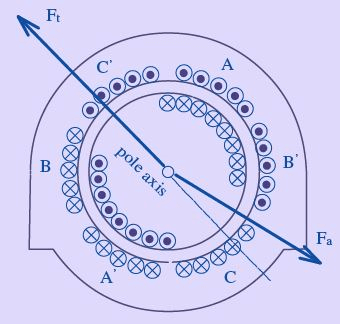Figure 22: Synchronous generator supplying a lagging pf load

convincingly divided into cross-magnetizing and direct-magnetizing components, since the uniform air-gap permits sinusoidal m.m.f s to produce more or less sinusoidal ﬂuxes. Fig. 22 shows a machine with two poles and the currents in the three-phase armature winding produce a reaction ﬁeld having a sinusoidally-distributed fundamental component and an axis coincident, for the instant considered, with that of one phase such as A − A′ . The rotor windings, energized by direct current, give also an approximately sinusoidal rotor m.m.f. distribution. The machine is shown in operation as a generator supplying a lagging current.

The relation of the armature reaction m.m.f. Fa to the ﬁeld m.m.f. Ft is shown in Fig. 23.

The Fa sine wave is resolved into the components Faq corresponding to the cross-component and Fad corresponding to the direct-component, which in this case demagnetizes in accordance with Fig. 20. Fad acts in direct opposition to Ft and reduces the eﬀective m.m.f. acting round the normal magnetic circuit. Faq shifts the axis of the resultant m.m.f. (and ﬂux) backward against the direction of rotation of the ﬁeld system.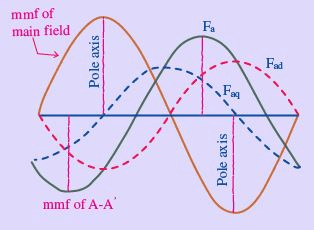Figure 23: Sinusoidal distribution of the components of armature reaction in a synchronous generator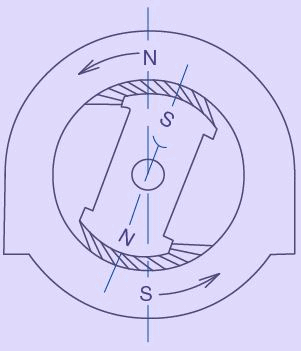Figure 24: Elementary synchronous motor action - Attraction of the unlike poles keep the rotor locked to the rotating ﬁeld produced in the stator

Behaviour of a loaded synchronous motor Likewise when a synchronous machine operates as a motor with a mechanical load on its shaft, it draws an alternating current which interacts with the main ﬂux to produce a driving torque. The torque remains unidirectional only if the rotor moves one pole-pitch per half-cycle; i.e. it can run only at the synchronous speed. In a balanced three-phase machine, the armature reaction due to the fundamental component of the current is a steady mmf revolving synchronously with the rotor - its constant cross-component producing a constant torque by interaction with the main ﬂux, while its direct-component aﬀects the amount of the main ﬂux. A very simple way of regarding a synchronous motor is illustrated in Fig. 24. The stator, like that of the induction motor produces a magnetic ﬁeld rotating at synchronous speed. The poles on the rotor (salient-pole is shown in Fig. 24 only for clarity), excited by direct current in their ﬁeld windings, undergo magnetic attraction by the stator poles, and are dragged round to align themselves and locked up with with the stator poles (of opposite polarity- obviously). On no load the axes of the stator and rotor poles are practically coincident. When a retarding torque is applied to the shaft, the rotor tends to fall behind. In doing so the attraction of the stator on the rotor becomes tangential to an extent suﬃcient to develop a counter torque - however the rotor continues to rotate only at synchronous speed.
The angular shift between the stator and rotor magnetic axes represents the torque (or load) angle (as shown later, in the phasor diagram). This angle naturally increases with the mechanical load on the shaft. The maximum possible load is that which retards the rotor so that the tangential attraction is a maximum. (It will be shown later that the maximum possible value for the torque angle is 90 electrical degrees - corresponding to a retardation of the rotor pole by one half of a pole pitch). If the load be increased above this amount, the rotor poles come under the inﬂuence of a like pole and the attraction between the stator and rotor poles ceases and the rotor comes to a stop. At this point we say that the synchronous motor pulled out of step. This situation arises much above the rated loads in any practical machine.

It is to be noted that the magnetic ﬁeld shown in Fig. 24 is only diagrammatic and for better understanding of the action of the synchronous machine - the ﬂux lines may be considered as elastic bands which will be stretched by application of the mechanical load on the shaft. Actually the ﬂux lines will enter or leave the stator and rotor surfaces nearly normally, on account of the high permeability of these members. In a salient-pole machine the torque is developed chieﬂy on the sides of the poles and on the sides of the teeth in a non-salient-pole machine.

Concept of Synchronous Reactance

The operation of the synchronous machine can be reduced to comparatively simple expression by the convenient concept of synchronous reactance. The resultant linkage of ﬂux with any phase of the armature of a synchronous machine is due, as has been seen, to the combined action of the ﬁeld and armature currents. For a simple treatment it is convenient to separate the resultant ﬂux into components: (a) the ﬁeld ﬂux due to the ﬁeld current alone; and (b) the armature ﬂux due to the armature current alone. This separation does not aﬀect qualitative matters, but its quantitative validity rests on the assumption that the magnetic circuit has a constant permeability. In brief the simplifying assumptions are: 1. The permeability of all parts of the magnetic circuit of the synchronous machine is constant - in other words the ﬁeld and armature ﬂuxes can be treated separately as proportional to their respective currents so that their eﬀects can be superposed. 2. The air gap is uniform, so that the armature ﬂux is not aﬀected by its position relative to the poles - in other words we assume the rotor to be cylindrical 3. The distribution of the ﬁeld ﬂux in the air gap is sinusoidal. 4. The armature winding is uniformly distributed and carries balanced sinusoidal currents.

In other words, the harmonics are neglected so that the armature ﬂux is directly proportional to the fundamental component of the armature reaction mmf implying that the armature reaction mmf is distributed sinusoidally and rotates at synchronous speed with constant magnitude.
Assumption (1) is roughly fulﬁlled when the machine works at low saturation; (2) and (3) are obviously inaccurate with salient-pole machines and assumption (4) is commonly made and introduces negligible error in most cases. The behaviour of an “ideal” synchronous machine can be indicated qualitatively when the above assumptions (1) to (4) are made.

The phasor diagrams Fig. 25 for the several conditions contain the phasors of two emfs viz. Eo and E . The latter is the e.m.f actually existing, while the former is that which would be induced under no-load conditions, i.e. with no armature current (or armature reaction).

Thus Eo is the e.m.f. corresponding to the ﬂux produced by the ﬁeld winding only, while E is that actually produced by the resultant ﬂux due to the combined eﬀect of stator and rotor ampere-turns. The actual e.m.f. E can be considered as Eo plus a ﬁctitious e.m.f. proportional to the armature current.

Fig. 25 is drawn in this manner with Er such that the following phasor relationship is satisﬁed:

E = Eo + Er             (19)

It can be seen from Fig. 25, that Er , is always in phase-quadrature with armature current and proportional to it (as per the four assumptions (1) to (4) above). The emf Er is thus similar to an emf induced in an inductive reactance, so that the eﬀect of armature reaction is exactly the same as if the armature windings had a reactance xa = Er /Ia . This ﬁctitious reactance xa can added to the armature leakage reactance xl and the combined reactance ( xa + x) is known as the synchronous reactance xs. The armature winding apart from these reactance eﬀects, presents a resistive behaviour also. Synchronous impedance is a tern used to denote the net impedance presented by each phase of the alternator winding, consisting of both resistive and reactive components. The behavior of a synchronous machine can be easily predicted from the equivalent circuit developed using this synchronous reactance xs, as explained in the following section.

Approximation of the Saturated Synchronous Reactance

Economical size requires the magnetic circuit to be somewhat saturated under normal operating conditions. However, the machine is unsaturated in the short-circuit test, and the synchronous reactance based on short-circuits and open-circuit test data is only an approximation at best. Nevertheless, there are many studies in which a value based on rated open-circuit voltage and the short circuit current suﬃces. Hence, in Fig. 29, if oc is rated voltage, ob is the required no-load ﬁeld current, which also produces the armature current o′e on short circuit. The synchronous impedance assuming the armature winding is star-connected is, accordingly,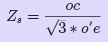20)

Except in very small machines, the synchronous reactance is much greater than the resistance (ra ) of the armature and the saturated value as well as the unsaturated value of the synchronous reactance and therefore is considered equal to the magnitude of the synchronous impedance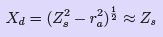(21)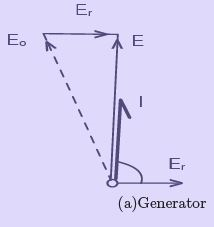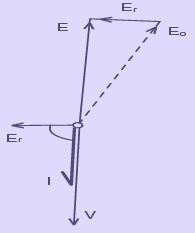(b)Motor unity power factor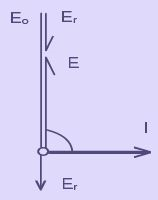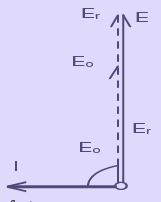(c) Generator                              (d)Generator zero power factor

Figure 25: Phasor diagrams for diﬀerent operating conditions

The line of in Fig. 29 is more nearly representative of the saturated machine than is the air-gap line. On the basis of this line, an estimate of the ﬁeld current can be obtained for a given terminal voltage, load current, and power factor. This is done by calculating Eaf and making use of the saturated synchronous reactance as follows.

Eaf = V + ZsI                        (22)

The ﬁeld current is that required to produce Eaf on the line of.

Open-circuit and Short-circuit Tests

The eﬀect of saturation on the performance of synchronous machines is taken into account by means of the magnetization curve and other data obtained by tests on an existing machine. Only some basic test methods are considered. The unsaturated synchronous impedance and approximate value of the saturated synchronous impedance can be obtained form the open-circuit and short-circuit tests.

In the case of a constant voltage source having constant impedance, the impedance can be found by dividing the open-circuit terminal voltage by the short circuit current.
However, when the impedance is a function of the open-circuit voltage, as it is when the machine is saturated, the open-circuit characteristic or magnetization curve in addition to the short-circuit characteristic is required.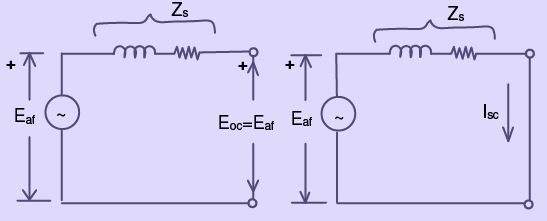Figure 26: Synchronous generator(a) Open circuit (b) Short circuit

The unsaturated synchronous reactance is constant because the reluctance of the unsaturated iron is negligible. The equivalent circuit of one phase of a polyphase synchronous machine is shown in Fig. 26 for the open-circuit condition and for the short circuit condition. Now Eaf is the same in both cases when the impedance Zs. Where Eaf is the open-circuit volts per phase and Isc is the short-circuit current per phase.

Open-circuit Characteristic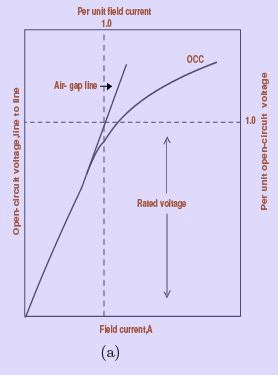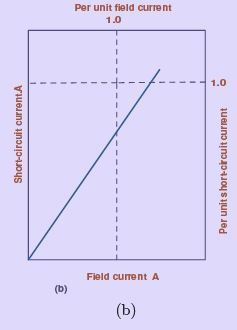Figure 27: (a) Open circuit characteristic and (b) Short-circuit characteristic

To obtain the open-circuit characteristic the machine is driven at its rated speed without load. Readings of line-to-line voltage are taken for various values of ﬁeld current. The voltage except in very low-voltage machines is stepped down by means of instrument potential transformers. Fig. 27 shows the open-circuit characteristic or no-load saturation curve. Two sets of scales are shown; one, line to-line volts versus ﬁeld current in amperes and the other per-unit open-circuit voltage versus per-unit ﬁeld current. If it were not for the magnetic saturation of the iron, the open-circuit characteristic would be linear as represented by the air-gap line in Fig. 27. It is important to note that 1.0 per unit ﬁeld current corresponds to the value of the ﬁeld current that would produce rated voltage if there were no saturation. On the basis of this convention, the per-unit representation is such as to make the air-gap lines of all synchronous machines identical.

Short circuit Test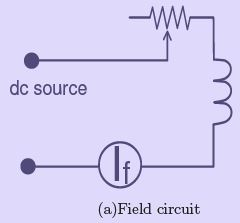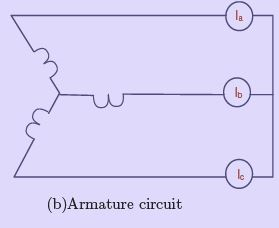Figure 28: Connections for short-circuit test

The three terminals of the armature are short -circuited each through a currentmeasuring circuit, which except for small machines is an instrument current transformer with an ammeter in its secondary. A diagram of connections in which the current transformers are omitted is shown in Fig. 28.

The machine is driven at approximately synchronous (rated) speed and measurements of armature short-circuit current are made for various values of ﬁeld current, usually up to and somewhat above rated armature current. The short-circuit characteristic (i.e. armature short circuit current versus ﬁeld current) is shown in Fig. 27. In conventional synchronous machines the short-circuit characteristic is practically linear because the iron is unsaturated up to rated armature current and somewhat beyond, because the magnetic axes of the armature and the ﬁeld practically coincide (if the armature had zero resistance the magnetic axes would be in exact alignment), and the ﬁeld and armature mmfs oppose each other.

Unsaturated Synchronous Impedance

The open circuit and short-circuit characteristics are represented on the same graph in Fig. 29. The ﬁeld current oa produces a line-to line voltage oc on the air- gap line, which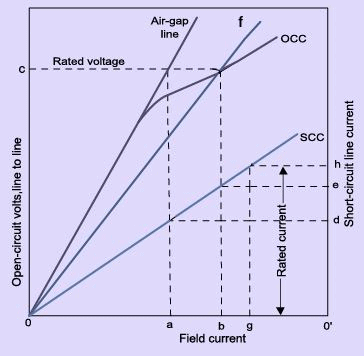Figure 29: Open-circuit and short circuit characteristic

would be the open-circuit voltage if there were no saturation. The same value of ﬁeld current produces the armature current o’d and the unsaturated synchronous reactance is given by: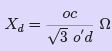phase, for a star connected armature                  (23)

When the open-circuit characteristic, air-gap line, and the short-circuit characteristic are plotted in per-unit, then the per unit value of unsaturated synchronous reactance equals the per-unit voltage on the air-gap line which results from the same value of ﬁeld current as that which produces rated short-circuit (one-per unit) armature current. In Fig. 29 this would be the per-unit value on the air gap line corresponding to the ﬁeld current og.

Offer running on EduRev: Apply code STAYHOME200 to get INR 200 off on our premium plan EduRev Infinity!

## Electrical Machines for Electrical Engg.

22 videos|41 docs|26 tests

,

,

,

,

,

,

,

,

,

,

,

,

,

,

,

,

,

,

,

,

,

;